# Partial Sums

A Partial Sum is a Sum of Part of a Sequence.

### Example:

This is the Sequence of even numbers from 2 onwards: {2, 4, 6, 8, 10, 12, ...}

This is the Partial Sum of the first 4 terms of that sequence: 2+4+6+8 = 20

Let us define things a little better now:

A Sequence is a set of things (usually numbers) that are in order.A Partial Sum is the sum of part of the sequence.

The sum of infinite terms is an Infinite Series.

And Partial Sums are sometimes called "Finite Series".

## Sigma

Partial Sums are often written using Σ to mean "add them all up":

Σ   This symbol (called Sigma) means "sum up"

So Σ means to sum things up ...

### Sum What?

Sum whatever is after the Sigma:

Σ

n
so we sum n

### But What Values of n ?

The values are shown below
and above the Sigma:

4
Σ
n=1
n
it says n goes from 1 to 4,
which is 1, 2, 3 and 4

### OK, Let's Go ...

So now we add up 1,2,3 and 4:

4
Σ
n=1
n = 1 + 2 + 3 + 4 = 10

Here it is in one diagram:## More Powerful

But Σ can do more powerful things than that!

We can square n each time and sum the result:
4
Σ
n=1
n2 = 12 + 22 + 32 + 42 = 30

We can add up the first four terms in the sequence 2n+1:

4
Σ
n=1
(2n+1) = 3 + 5 + 7 + 9 = 24

And we can use other letters, here we use i and sum up i × (i+1), going from 1 to 3:

3
Σ
i=1
i(i+1) = 1×2 + 2×3 + 3×4 = 20

And we can start and end with any number. Here we go from 3 to 5:

5
Σ
i=3
ii + 1 = 34 + 45 + 56

## Properties

Partial Sums have some useful properties that can help us do the calculations.

### Multiplying by a Constant Property

Say we have something we want to sum up, let's call it ak

ak could be k2, or k(k-7)+2, or ... anything really

And c is some constant value (like 2, or -9.1, etc), then: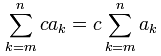In other words: if every term we are summing is multiplied by a constant, we can "pull" the constant outside the sigma.

### Example: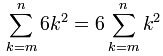So instead of summing 6k2 we can sum k2 and then multiply the whole result by 6

Here is another useful fact: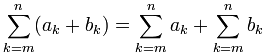Which means that when two terms are added together, and we want to sum them up, we can actually sum them separately and then add the results.

### Example: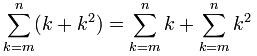It is going to be easier to do the two sums and then add them at the end.

Note this also works for subtraction: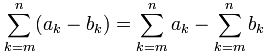## Useful Shortcuts

And here are some useful shortcuts that make the sums a lot easier.

In each case we are trying to sum from 1 to some value n.Summing 1 equals nSumming the constant c equals c times nA shortcut when summing kA shortcut when summing k2A shortcut when summing k3Also true when summing k3Summing odd numbers

Let's use some of those:

### Example 1: You sell concrete blocks for landscaping.

A customer says they will buy the entire "pyramid" of blocks you keep out front. The stack is 14 blocks high.

How many blocks are in there?Each layer is a square, so the calculation is:

12 + 22 + 32 + ... + 142

But this can be written much more easily as: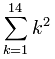We can use the formula for k2 from above: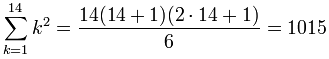That was a lot easier than adding up 12 + 22 + 32 + ... + 142.

And here is a more complicated example:

### Example 2: The customer wants a better price.

The customer says the blocks on the outside of the pyramid should be cheaper, as they need cleaning.

You agree to:

• \$7 for outer blocks
• and \$11 for inner blocks.

What is the total cost?You can calculate how many "inner" and "outer" blocks in any layer (except the first) using

• outer blocks = 4×(size-1)
• inner blocks = (size-2)2

And so the cost per layer is:

• cost (outer blocks) = \$7 × 4(size-1)
• cost (inner blocks) = \$11 × (size-2)2

So all layers together (except first) will cost: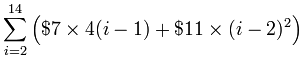Now we have the sum, let us try to make the calculations easier!

Using the "Addition Property" from above: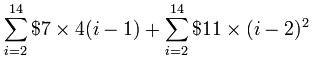Using the "Multiply by Constant Property" from above:That is good ... but we can't use any shortcuts as it is, as we are going from i=2 instead of i=1

HOWEVER, if we invent two new variables:

• j = i-1
• k = i-2

We have:(I dropped the k=0 case, because I know that 02=0)

And now we can use the shortcuts: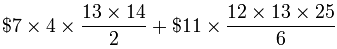After a little calculation:

\$7 × 364 + \$11 × 650 = \$9,698.00

Oh! And don't forget the top layer (size=1) which is just one block. Maybe you can give them that one for free, you are so generous!

Note: as a check, when we add the "outer" and "inner" blocks, plus the one on top, we get

364 + 650 + 1 = 1015

Which is the same number we got for the "total blocks" before ... yay!